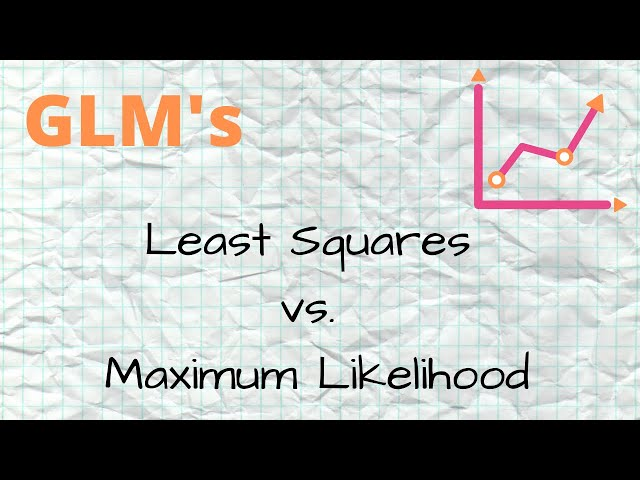# What is Generalized linear models

##### Understanding Generalized Linear Models: A Comprehensive Guide
Introduction

Generalized Linear Models (GLMs) are one of the many types of statistical models used to predict outcomes from a given dataset. Unlike classical linear models, GLMs allows for the modeling of discrete and/or continuous random variables that are independent of one another. It is widely used not just in scientific communities but also in various industries such as healthcare, finance, and agriculture.

In this comprehensive guide, we will discuss all the basic concepts and types of GLMs you need to learn as an AI expert. We will also explain how GLMs can be implemented, its advantages and disadvantages, and some real-world applications.

What is a Generalized Linear Model (GLM)?

GLMs can be defined as an extension of Linear Models (LMs) for predicting the relationship between the outcome variable and one or more predictor variables. It combines classical linear models with some non-linear functions to allow for non-normal error distribution and non-constant variance of the dependent variable.

LMs are efficient and widely used in many statistical applications. However several data distributions cannot be modeled using linear regression. Therefore, if the response variable is not normally distributed, classical linear models might give false inferences. GLMs offer an alternative method for modeling such data sets.

GLMs are particularly useful when trying to model common negative binomial responses such as count data. Depending on the situation, there are different types of GLMs that can be used that are discussed below.

##### Types of GLMs
• 1. Gaussian Family with Identity Link: This method assumes that normal distribution is a good fit for the data. It is used for continuous variables to estimate the mean and variance of these data. The identity function can be used to link responses to predictors.
• 2. Binomial Family with Logit Link: This is commonly used when the outcome variable is binary: true/false or 0/1. The logit link function maps the probability of the response variable to a linear combination of predictors.
• 3. Poisson Family with Log Link: Poisson regression models are useful when the response variable is a count of events over some fixed period. It can also be used to model the occurrence of rare events in some given time. The log link function can be used to fit these models.
• 4. Beta Family with Logit Link: Beta regression models are used when the response variable has a limited range where variables can become negative or if their values are between 0 and 1. Here, the logit link function maps values in (0,1) to a linear combination of predictors.
##### GLMs have a wide range of advantages when compared to other statistical models. They include: Ease in handling non-normal data, making them usable for a wider range of experiments Handles crossover points and interaction well The modeling method is widely understood, making it easy to interpret results. Models can be customized to conform to specific data sets and situations

When working with GLMs, there are some potential drawbacks. They include:

• They are not as widely known or understood as some other types of statistical models, making them more challenging to work with in some settings.
• Overfitting may occur where the model becomes too complex and does not accurately generalize the data.
• Underfitting may occur when the model is too simple and fails to capture important details in the data, resulting in less predictive power.
##### Applications of GLMs in the real world

GLMs are widely implemented in various industries and fields of science. Here are a few real-world applications:

• Finance – GLMs are useful tools for predicting the probability of different types of financial crises or risk models.
• Marketing and Advertising – GLMs are effective in predicting and forecasting marketing trends or the purchase propensity of different demographic groups.
• Healthcare – GLMs are helpful in modeling the relationship between different treatments or interventions to specific disease outcomes.
• Environmental Sciences – GLMs are good models for predicting the impact of different weather and environmental conditions on different species over time.
• Social Sciences – GLMs can be used to model different outcomes for different groups of people in social science experiments.
##### Conclusion

Generalized Linear Models are powerful statistical models used to analyze data in various fields. They are used to predict outcomes, model relationships between different variables, and explore data patterns where classical linear regression models are inadequate. As an AI expert, understanding the fundamentals of GLMs and its usage procedures are essential in creating and analyzing complex data sets efficiently.